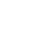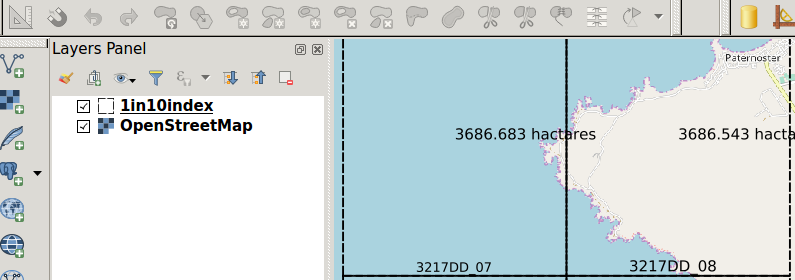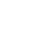### Labelling with geometry expressions in Context

“Expressions facilitates the manipulation of attribute values and geometry of layers. Expressions can also be used to calculate labels on the fly.”

The geometry functions are a special type of functions which are used to manipulate the geometry of layers. The geometry functions can be used in multiple places like labelling and symbology. When the geometry expressions are used in labelling they act like virtual fields/virtual layers where they evaluate the expressions on the fly and render them independent of the attribute table.
In this module, we will look at how we can use the geometry functions to generate labels.#### You try:

Goal: To learn how to use the geometry functions to label polygons

• Load the exercise data
• Symbolise the vector layer using the values defined in the table.
• Add a background layer i.e web map or any XYZ tile layers and ensure the rendering order has the raster layer at the bottom.
• Go to label settings and add a rule-based label.
• Use the Area label expression to label the layer.
• Play around with the placement setting and determine if you can place the label at the centre of the polygon.
• In the placement properties use data defined settings and use the expression X and Y .
• Add another second rule and label with the location expression.
• On the placement use 'Perimeter'.

Name Expectation

Vector style

Fill type = transparent, outline style =dash line

Area label

round( area(transform( \$geometry ,'EPSG:4326','EPSG:3857')) / 10000,3)

location

substr( location,1,6 ) || ' ' || substr( location,8,8)

X

x(centroid( \$geometry))

Y

y(centroid( \$geometry))### More about labelling using geometry expressions

Geometry functions are very useful as they allow on the fly computation of values. This reduces the need to have to create multiple layers or attributes to hold certain values. When using the geometry functions in labelling you have to take into consideration the number of features that are in the layer as this tends to make it slow.

Example: x(line_interpolate_point(\$geometry,0.7*\$length))
The function above places a label at 70 % of the length of the line.### Check your knowledge:

1. What is a geometry function:
1. A type of vector data.
2. A type of symbology mechanism that can be used for rasters and vector layers.
3. A type of function that allows new values to be computed based on existing geometry of the layer.
2. When using the geometry functions to calculate area does the data need to be in a projected co ordinate reference system:
1. Yes, you can only get accurate area interpretation when the data is projected.
2. No, A GIS should be able to handle this.
3. I do not know.
3. The units of area can only be metres.:
1. True
2. False